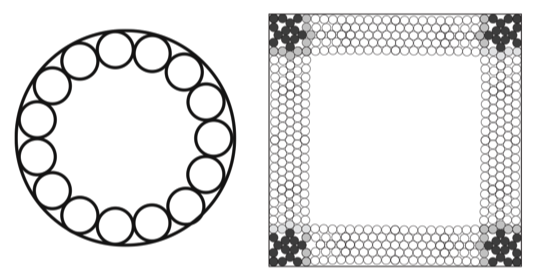# Name and Algorithms for a Sparsest Circle Packing

The ordinary circle packing problem in the variant with equal radii asks for the largest radius $$r_{max}$$ that allows placing $$n$$ non-overlapping circles with radius $$r_{max}$$ e.g. in the unit square, in the unit circle or, on the unit sphere (Tammes' problem).

Now, I would like to solve a somehow opposite problem:

Question:

given a number $$n\in\mathbb{N}$$, what is the smallest radius $$r_{min}\in\mathbb{R}^+$$ that permits a non-overlapping, rigid placement of $$n$$ circles with radius $$r_{min}$$ in the unit square, or in the unit circle or, on the unit sphere?

Under a rigid configuration I understand a configuration, where every open halfplane, resp. hemisphere defined by a hyperplane through a circle's center contains at least one contact point with another circle or, with the boundary of the containing region.

Are there already algorithms and/or theoretical results available for that problem?

• To make it clear, you might illustrate with a penny packing and with a packing where "the central pennies are removed". In particular, the Pack-o-mania website might talk about rigid packing already. Gerhard "Some Holes In My Remembering" Paseman, 2018.08.11. – Gerhard Paseman Aug 11 '18 at 15:57
• @GerhardPaseman removing pennies from a packing of course yields a rigid packing, however not necessarily the sparsest possible. – Manfred Weis Aug 11 '18 at 16:03
• I am not sure about the added note in the OP that in the square arrangement "certain coins can be moved orthogonally to a side of the square"; notice that the rows of discs in the lower right figure of my answer have a slight curvature, so the discs are kept in place in the same way as for the packing in the circle. I thought that was the whole point of this structure, these five lines of discs along each boundary of the square have a curvature that keeps each disc fixed in place by its neighbors. – Carlo Beenakker Aug 12 '18 at 13:24
• I didn't notice the curvature in the image; I will check what I overlooked. – Manfred Weis Aug 12 '18 at 13:59
• After checking the article, I see that it indeed also solves the problem in case of the unit squares. I will remove the addendum. – Manfred Weis Aug 12 '18 at 15:20

An overview of this "sparse packing problem" is given by Matthew Kahle in Sparse locally-jammed disk packings. Quite generally, $r_{\rm min}=O(1/n)$, so the fraction of the unit square or unit disc covered by the $n$ circles goes to 0 as their number $n$ goes to infinity. In a disc you just line up the circles along the perimeter, and this works for any smooth boundary. For a polygon, such as a square, the construction is more involved to avoid loose circles at the corners.• that is a good pointer for further search; I'm only missing the solution, if the number $n$ of circles is given. – Manfred Weis Aug 11 '18 at 19:33
• if $n$ is given and you can vary $r$, can't you just reduce $r$ until the circles can be lined up along the perimeter of the disc? – Carlo Beenakker Aug 11 '18 at 19:38